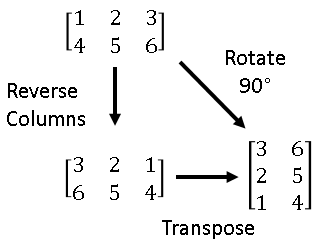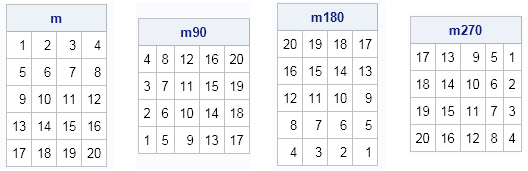No, I don't mean "rotation matrices," I mean rotating matrices. As in turning a matrix 90 degrees in a clockwise or counterclockwise direction.

I was reading a program written in MATLAB in which the programmer used a MATLAB function called ROT90, which rotates a matrix counterclockwise by multiples of 90 degrees. I thought it would be fun to implement that MATLAB function in the SAS/IML language.I wasn't sure of the most efficient way to implement the function. It seems like it might require writing a loop. But then I realized that rotating a matrix can be accomplished by using three simple "flip" operations: diagonal flips, up-down flips, and left-right flips. A flip across the diagonal is the matrix transpose operation. An up-down flip is equivalent to reversing the rows of a matrix. The left-right flip is accomplished by reversing the columns of a matrix. Each of these operations is easy to implement, and by composing two operations you can obtain a rotation of the elements of the matrix.

For example, the adjacent diagram shows that rotating a matrix by 90 degrees is equivalent to reversing its columns, followed by transposing the matrix. (Equivalently, you could transpose and then reverse the rows.) In the same way, you can rotate a matrix by 270 degrees by reversing the rows and then transposing. Lastly, you can rotate a matrix by 180 degrees by reversing the rows and the columns. Consequently, rotating a matrix is accomplished by the following one-line SAS/IML functions:

```proc iml; start Rot90(m); return( T(m[,ncol(m):1]) ); /* left-right flip, then transpose */ finish;   start Rot180(m); return( m[nrow(m):1,ncol(m):1] ); /* left-right flip, up-down flip */ finish;   start Rot270(m); return( T(m[nrow(m):1,]) ); /* up-down flip, then transpose */ finish;   m = shape(1:20,5); m90 = rot90(m); m180 = rot180(m); m270 = rot270(m); print m m90 m180 m270;```The implementation of the functions shows that it is sometimes possible to generate a complicated transformation by considering products of simpler transformations. I have previously used the relationship between flips and rotations to demonstrate how to use matrix operations to extend the life of your bed mattress. Whether you are rotating mattresses or matrices, the task is made easier by using geometry and the SAS/IML language.

Share